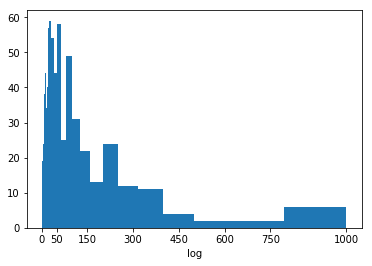# Python：matplotlib 之标尺和变换 (三十一)

## 标尺和变换

plt.figure(figsize = [10, 5])

# histogram on left: natural units
plt.subplot(1, 2, 1)
bin_edges = np.arange(0, ln_data.max()+100, 100)
plt.hist(ln_data, bins = bin_edges)

# histogram on right: directly log-transform data
plt.subplot(1, 2, 2)
log_ln_data = np.log10(ln_data)
log_bin_edges = np.arange(0.8, log_ln_data.max()+0.1, 0.1)
plt.hist(log_ln_data, bins = log_bin_edges)
plt.xlabel('log(values)') # add axis label for clarity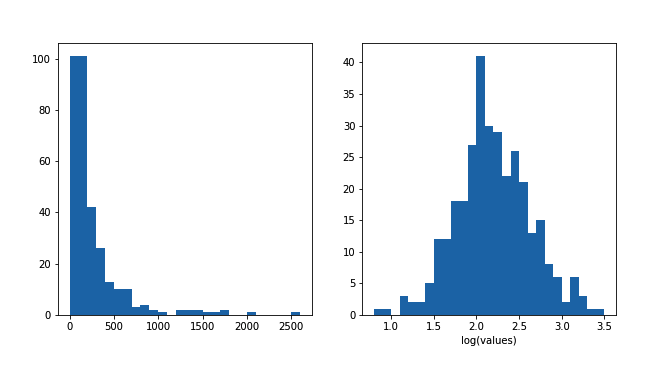bin_edges = np.arange(0, ln_data.max()+100, 100)
plt.hist(ln_data, bins = bin_edges)
plt.xscale('log')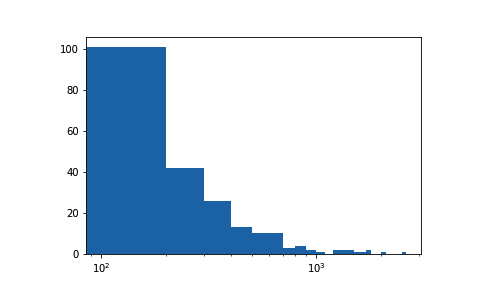bin_edges = 10 ** np.arange(0.8, np.log10(ln_data.max())+0.1, 0.1)
plt.hist(ln_data, bins = bin_edges)
plt.xscale('log')
tick_locs = [10, 30, 100, 300, 1000, 3000]
plt.xticks(tick_locs, tick_locs)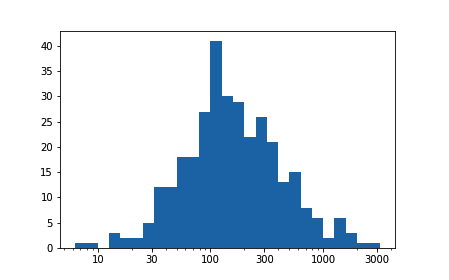## 替代方法

def sqrt_trans(x, inverse = False):
""" transformation helper function """
if not inverse:
return np.sqrt(x)
else:
return x ** 2

bin_edges = np.arange(0, sqrt_trans(ln_data.max())+1, 1)
plt.hist(ln_data.apply(sqrt_trans), bins = bin_edges)
tick_locs = np.arange(0, sqrt_trans(ln_data.max())+10, 10)
plt.xticks(tick_locs, sqrt_trans(tick_locs, inverse = True).astype(int))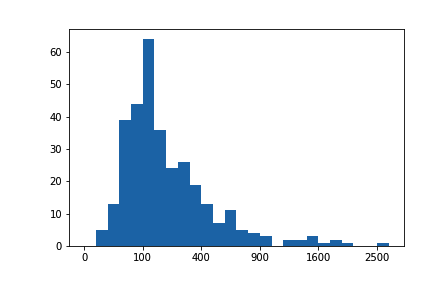## 练习

# prerequisite package imports
import numpy as np
import pandas as pd
import matplotlib.pyplot as plt
import seaborn as sb

%matplotlib inline

from solutions_univ import scales_solution_1, scales_solution_2

pokemon = pd.read_csv('./data/pokemon.csv')
pokemon.head()
id species generation_id height weight base_experience type_1 type_2 hp attack defense speed special-attack special-defense
0 1 bulbasaur 1 0.7 6.9 64 grass poison 45 49 49 45 65 65
1 2 ivysaur 1 1.0 13.0 142 grass poison 60 62 63 60 80 80
2 3 venusaur 1 2.0 100.0 236 grass poison 80 82 83 80 100 100
3 4 charmander 1 0.6 8.5 62 fire NaN 39 52 43 65 60 50
4 5 charmeleon 1 1.1 19.0 142 fire NaN 58 64 58 80 80 65

# YOUR CODE HERE
bin_edges = np.arange(0, pokemon['height'].max() + 0.2, 0.2)
#print(bin_edges)
plt.hist(data=pokemon, x='height', bins=bin_edges)
plt.xlim(0,6)
(0, 6)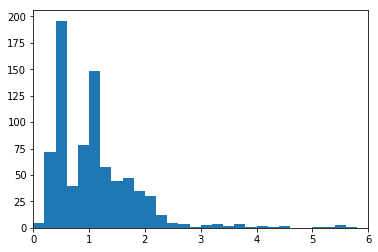# run this cell to check your work against ours
scales_solution_1()
There's a very long tail of Pokemon heights. Here, I've focused in on Pokemon of height 6 meters or less, so that I can use a smaller bin size to get a more detailed look at the main data distribution.# YOUR CODE HERE
log_ln_data = np.log10(pokemon['weight'])
log_bin_edges = np.arange(0, log_ln_data.max()+0.1, 0.1)
plt.hist(log_ln_data, bins = log_bin_edges)
plt.xlabel('log(values)') # add axis label for clarity
Text(0.5,0,'log(values)')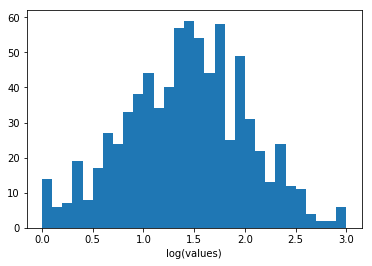# run this cell to check your work against ours
scales_solution_2()
Since Pokemon weights are so skewed, I used a log transformation on the x-axis. Bin edges are in increments of 0.1 powers of ten, with custom tick marks to demonstrate the log scaling.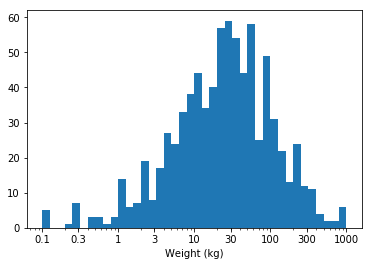# YOUR CODE HERE
bin_edges = 10 ** np.arange(0, log_ln_data.max()+0.1, 0.1)
plt.hist(pokemon['weight'], bins = bin_edges)
plt.xlabel('log') # add axis label for clarity
tick_locs = [0, 50, 150, 300, 450, 600, 750, 1000]
plt.xticks(tick_locs, tick_locs)
([<matplotlib.axis.XTick at 0x7f5489ec5cf8>,
<matplotlib.axis.XTick at 0x7f5489ec57b8>,
<matplotlib.axis.XTick at 0x7f5489e4e470>,
<matplotlib.axis.XTick at 0x7f5489e37e48>,
<matplotlib.axis.XTick at 0x7f5489e3f4e0>,
<matplotlib.axis.XTick at 0x7f5489e3fb38>,
<matplotlib.axis.XTick at 0x7f5489e441d0>,
<matplotlib.axis.XTick at 0x7f5489e44860>],
<a list of 8 Text xticklabel objects>)